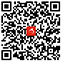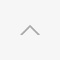## 黄金成色指什么

8k=8*4.166%=33.328%(333‰)

9k=9*4.166%=37.494%(375‰)

10k=10*4.166%=41.660%(417‰)

12k=12*4.166%=49.992%(500‰)

14k=14*4.166%=58.324%(583‰)

18k=18*4.166%=74.998%(750‰)

20k=20*4.166%=83.320%(833‰)

21k=21*4.166%=87.486%(875‰)

22k=22*4.166%=91.652%(916‰)

24k=24*4.166%=99.984%(999‰)

• ### 黄金图集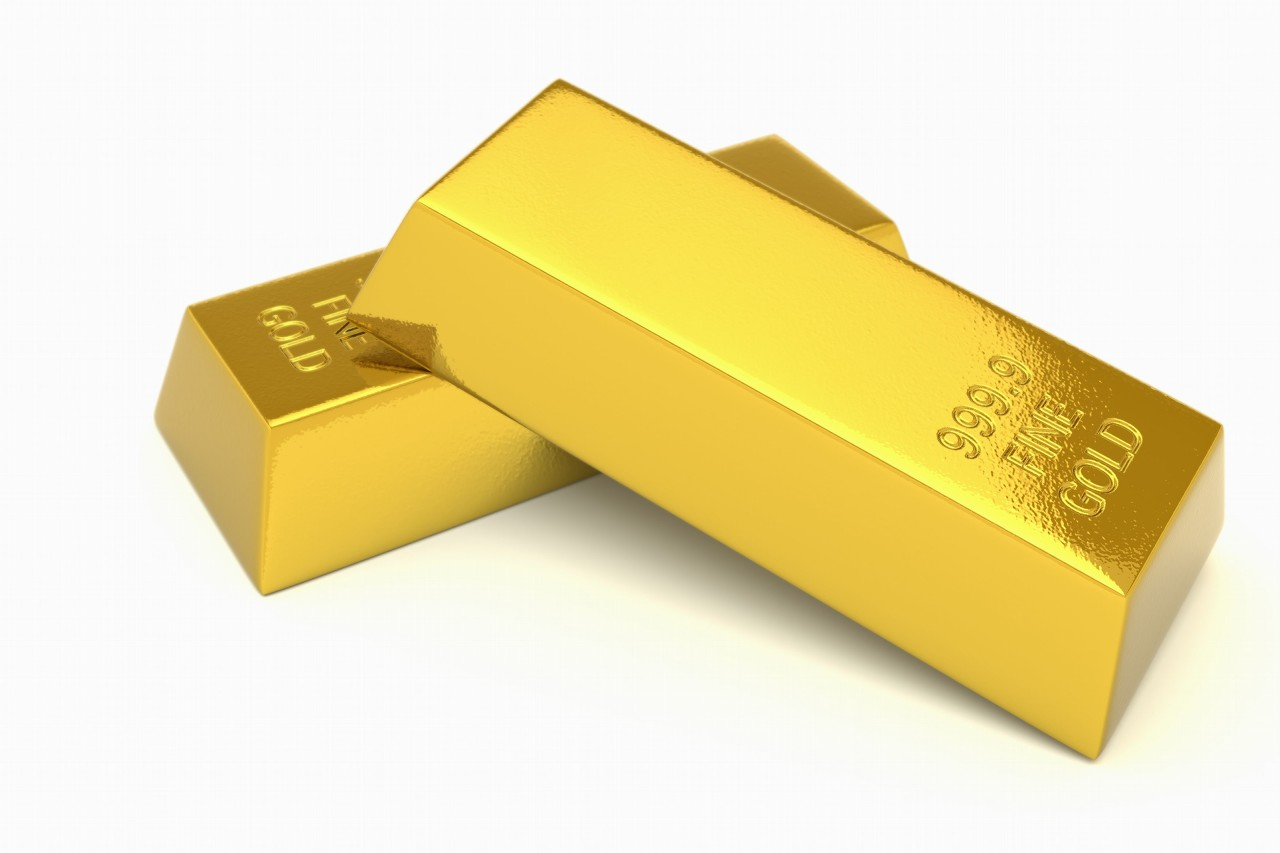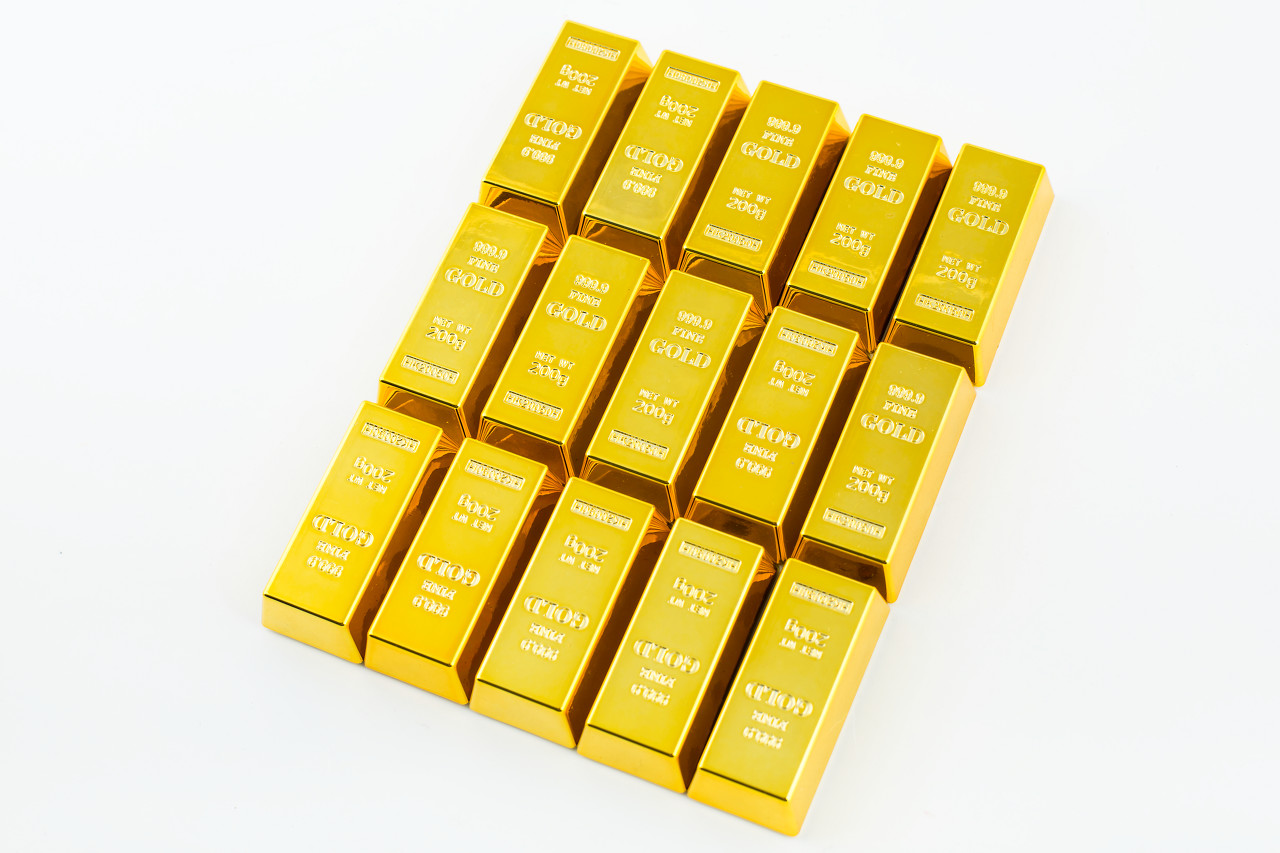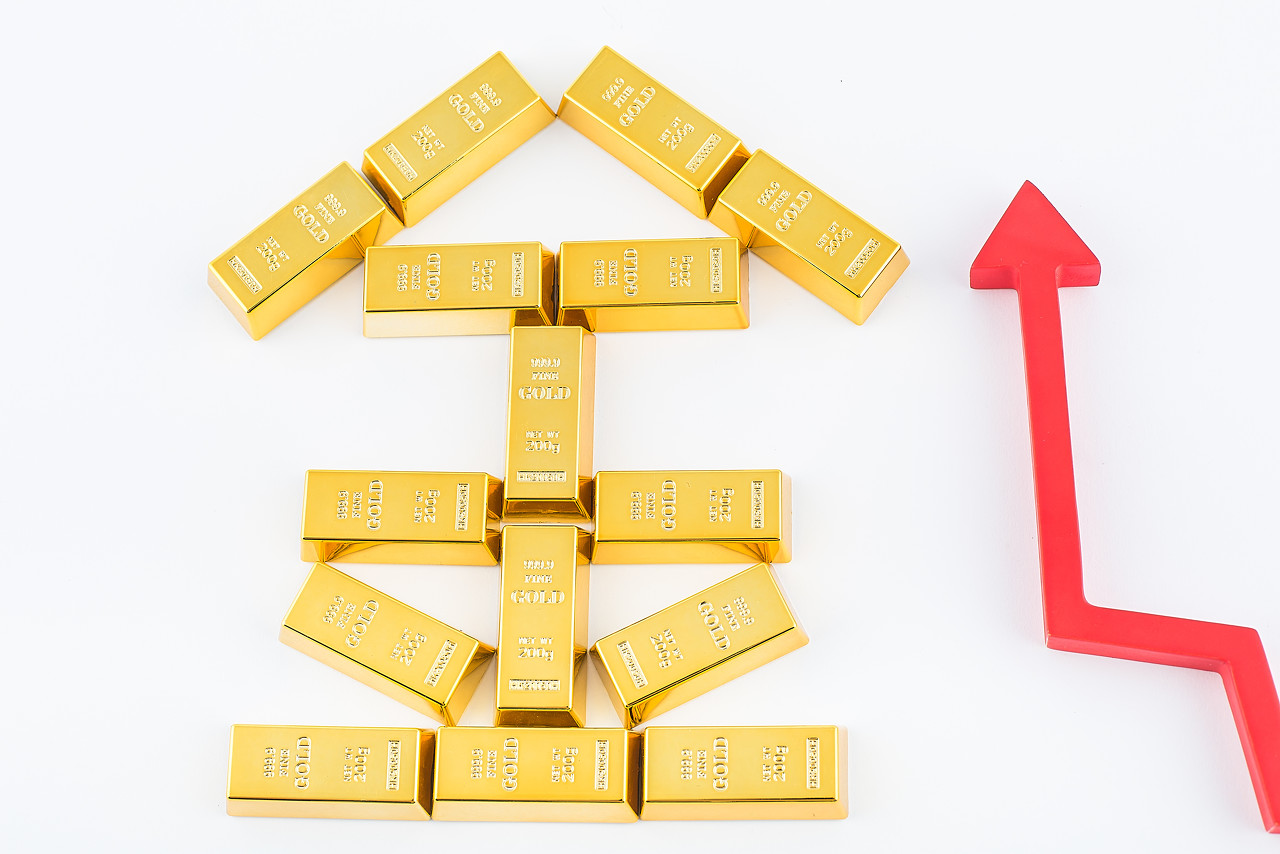2017年英国大选最终以悬持议会的出现落下了帷幕...